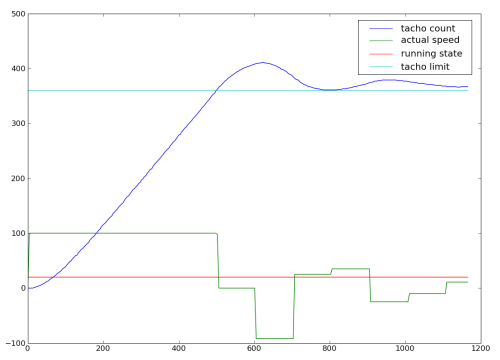# nxt memory access

In attempt to improve the motor rotation, I needed a way to trace data coming from the NXT. I noticed that the virtual machine memory was accessible from IO map, so any program variable can be extracted from a remote computer (through USB or bluetooth).

Here is the idea: record information in an array and download the array to the computer. The NXC program collecting data:

```/* Number of measure points. */
#define MEASURE_SIZE 2048

/* Measure points. */
unsigned long measure[MEASURE_SIZE];

/* Set to one to measure. */
int measure_go = 0;

/* Measure task, will trace when measure_go is set. */
{
unsigned long last, new = 0;
int i = 0;
while (1)
{
if (measure_go)
{
/* Wait until the date change. */
last = new;
do
new = CurrentTick ()
while (last == new);
/* Record date and values. */
measure[i] = new << 24
| (MotorRunState (OUT_A) & 0xf0) << 16
| (MotorActualSpeed (OUT_A) & 0xff) << 12
| MotorTachoCount (OUT_A) & 0xfff;
/* Update trace index. */
i = (i + 1) % MEASURE_SIZE;
}
}
}
```

Here, several pieces of information are stored in the same measure word, but other strategies could be used.

Now, how to retrieve information? Every variables in the NXT virtual machine are identified with a data segment identifier which is directly used as an index in the data segment. For simple variables, value is directly stored at this index. For dynamic variables like arrays, the stored value is an index in the DOPE vector array which can also be read using IO map.

When you compile a NXC program you can ask for the symbols file, here is lines concerning my measure array:

``````#SYMBOLS
Index   Identifier      Type    Flag    Data    Size    RefCount
[...]
95      measure 7       0       184     4       3
96      measure.measure_type    5       0       0       4       0
``````

The interesting number is 184, this is the data segment identifier.

OK, let's have a look to NXT firmware code. This is the CMD module IO map (CMD module implements the virtual machine):

```typedef struct
{
UBYTE FormatString[VM_FORMAT_STRING_SIZE];
UWORD (*pRCHandler)(UBYTE *, UBYTE *, UBYTE *);
ULONG Tick;
UWORD OffsetDS;
UWORD OffsetDVA;
PROGRAM_STATUS ProgStatus;
UBYTE Awake;
UBYTE ActivateFlag;
UBYTE DeactivateFlag;
UBYTE FileName[FILENAME_LENGTH + 1];
ULONG MemoryPool[POOL_MAX_SIZE / 4];
} IOMAPCMD;
```

Interesting fields are:

• MemoryPool: this is all the data allocated by the virtual machine.
• OffsetDS: start of data segment in MemoryPool, indexed from the IO map start.
• OffsetDVA: start of DOPE vector array in MemoryPool, indexed the same way.

Now the DOPE vector:

```typedef struct
{
UWORD Offset;
UWORD ElemSize;
UWORD Count;
UWORD BackPtr;
} DOPE_VECTOR;
```

Interesting fields are:

• Offset: current offset of the array inside the data segment.
• ElemSize: size of each array element.
• Count: current number of elements.

OK, we have enough information to retrieve all this. I use NXT-Python but other library could be used:

```import nxt.locator
import nxt.brick
import sys
from struct import unpack

def get_variable_id (file, *variables):
'''Read NBC symbol file to extract variables identifiers.'''
found = { }
f = open (file)
for line in f:
if line == '#SOURCES\n':
break
if line != '#SYMBOLS\n':
index, name, type, flag, id, size, refcount = line.split ('\t')
if name in variables:
found[name] = int (id)
return tuple (found[name] for name in variables)

def dump (b, id, count, elem_size):
'''Dump an array from NXT memory.'''
mod_id, n_bytes, contents = b.read_io_map (0x00010001, 16 + 4 + 4, 4)
offset_ds, offset_dva = unpack ('<2H', contents)
# Get DV index.
mod_id, n_bytes, contents = b.read_io_map (0x00010001, offset_ds + id, 2)
dv_index, = unpack ('<H', contents)
# Get DOPE vector.
mod_id, n_bytes, contents = b.read_io_map (0x00010001, offset_dva + 10 * dv_index, 6)
offset, r_elem_size, r_count = unpack ('<3H', contents)
assert count == r_count
assert elem_size == r_elem_size
result = []
for i in xrange (0, count * elem_size, 32):
mod_id, n_bytes, contents = b.read_io_map (0x00010001, offset_ds + offset + i, 32)
assert elem_size == 4
result += unpack ('<8I', contents)
# Print result.
print '\n'.join ('0x%08x' % r for r in result)

id, = get_variable_id ('tracerotate.sym', 'measure')

sock = nxt.locator.find_one_brick ()
if sock:
dump (sock.connect (), id, 2048, 4)
sock.close ()
```

This program is still rather crude, I should add command line options instead of hard coded values.

Now a plot program, also using python. This is not really part of the technique, but it might be useful:

```import sys
import matplotlib.pylab as p

x = [ ]
y = [ ]
stop = 360

lastdate = None
offset = 0
for line in sys.stdin:
i = int (line, 16)
# Take date.
date = (i >> 24) & 0xff
if lastdate is None:
offset = date
date = 0
else:
# Handle date rollover.
date = lastdate >> 8 << 8 | date
date -= offset
if date < lastdate:
date += 0x100
lastdate = date
# Append to data to plot.
x.append (date)
tacho = i & 0xfff
if tacho > 0x800:
tacho = -(0x1000 - tacho)
out = (i >> 12) & 0xff
if out > 0x80:
out = -(0x100 - out)
state = (i >> 20) & 0xf
y.append ((tacho, out, state * 10))
# Plot.
p.plot (x, y, (x, x[-1]), (stop, stop))
p.legend (('tacho count', 'actual speed', 'running state', 'tacho limit'))
p.show ()
```

Here is the result for a RotateMotor:The 100 ms NXT motor control period is clearly shown, but this is another story.